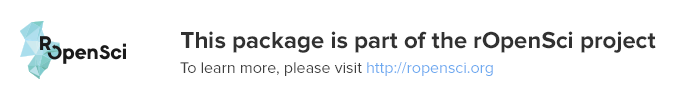# plotly 0,3Create interactive web graphics from R via plotly's JavaScript graphing library# plotly

An R package for creating interactive web graphics via the open source JavaScript graphing library plotly.js.

## Installation

Install from CRAN:

``````install.packages("plotly")
``````

Or install the latest development version (on GitHub) via devtools:

``````devtools::install_github("ropensci/plotly")
``````

## Getting Started

### Web-based ggplot2 graphics

If you use ggplot2, `ggplotly()` converts your plots to an interactive, web-based version! It also provides sensible tooltips, which assists decoding of values encoded as visual properties in the plot.

``````library(plotly)
g <- ggplot(faithful, aes(x = eruptions, y = waiting)) +
stat_density_2d(aes(fill = ..level..), geom = "polygon") +
xlim(1, 6) + ylim(40, 100)
ggplotly(g)
``````If you’d like to see how `ggplotly()` does in converting different ggplot2 examples, we host a plotly version of the official ggplot2 documentation. We also have some of our own examples here.

### plotly’s custom R interface

plotly.js supports some chart types that ggplot2 doesn’t (our cheatsheet provides a nice summary of the available chart types). You can create any of these charts via `plot_ly()`.

``````plot_ly(z = ~volcano, type = "surface")
``````We have a number of vignettes which explain the `plot_ly()` interface in depth as well as numerous examples on the plotly website and bundled with the package.

### Capturing plotly events

plotly.js exposes a number of ‘standard’ events that work consistently across plot types. It’s easy to hook into these events using the `event_data()` function in shiny apps, as these examples demonstrate:You can also hook into these events without shiny using `htmlwidgets::onRender()` (example). This, however, requires JavaScript knowledge and makes it much harder to coordinate views between htmlwidgets.

We have lots of examples on https://plot.ly/r/ and https://plot.ly/ggplot2/, but a more comprehensive review is also available at https://cpsievert.github.io/plotly_book/

## Contributing

Please read through our contributing guidelines. Included are directions for opening issues, asking questions, contributing changes to plotly, and our code of conduct.### Related Repositories

#### plotly.js

The open source JavaScript graphing library that powers plotly ...

#### plotly.py

An interactive, browser-based graphing library for Python :sparkles: ...

#### plotly

Create interactive web graphics from R via plotly's JavaScript graphing library ...

#### IPython-plotly

A collection of data science IPython notebooks with Plotly graphs ...

#### arduino-api

Arduino library for real-time logging and streaming data to online plotly graphs ...

### Releases

-   v4.5.2 zip tar
-   v3.6.1 zip tar
-   v3.6.0 zip tar
-   v3.5.5 zip tar
-   v3.5.4 zip tar
-   v3.5.3 zip tar
-   v3.5.2 zip tar
-   v3.5.1 zip tar
-   v3.4.13 zip tar
-   0.3 zip tar